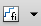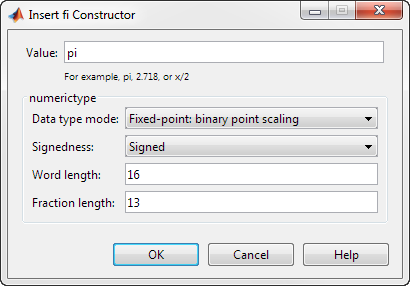## Ways to Construct fi Objects

### Types of fi Constructors

You can create `fi` objects using Fixed-Point Designer™ software in any of the following ways:

• You can use the `fi` constructor function to create a `fi` object.

• You can use the `sfi` constructor function to create a new signed `fi` object.

• You can use the `ufi` constructor function to create a new unsigned `fi` object.

• You can use any of the `fi` constructor functions to copy an existing `fi` object.

To get started, to create a `fi` object with the default data type and a value of 0:

```a = fi(0) ```
```a = 0 DataTypeMode: Fixed-point: binary point scaling Signedness: Signed WordLength: 16 FractionLength: 15 ```

This constructor syntax creates a signed `fi` object with a value of 0, word length of 16 bits, and fraction length of 15 bits. Because you did not specify any `fimath` object properties in the `fi` constructor, the resulting `fi` object `a` has no local `fimath`.

To see all of the `fi`, `sfi`, and `ufi` constructor syntaxes, refer to the respective reference pages.

For information on the display format of `fi` objects, refer to View Fixed-Point Data.

### Examples of Constructing fi Objects

The following examples show you several different ways to construct `fi` objects. For other, more basic examples of constructing `fi` objects, see the Examples section of the following constructor function reference pages:

Note

The `fi` constructor creates the `fi` object using a `RoundingMethod` of `Nearest` and an `OverflowAction` of `Saturate`. If you construct a `fi` from floating-point values, the default `RoundingMethod` and `OverflowAction` property settings are not used.

#### Constructing a fi Object with Property Name/Property Value Pairs

You can use property name/property value pairs to set `fi` and `fimath` object properties when you create the `fi` object:

```a = fi(pi, 'RoundingMethod','Floor', 'OverflowAction','Wrap') ```
```a = 3.1415 DataTypeMode: Fixed-point: binary point scaling Signedness: Signed WordLength: 16 FractionLength: 13 RoundingMethod: Floor OverflowAction: Wrap ProductMode: FullPrecision SumMode: FullPrecision```

You do not have to specify every `fimath` object property in the `fi` constructor. The `fi` object uses default values for all unspecified `fimath` object properties.

• If you specify at least one `fimath` object property in the `fi` constructor, the `fi` object has a local `fimath` object. The `fi` object uses default values for the remaining unspecified `fimath` object properties.

• If you do not specify any `fimath` object properties in the `fi` object constructor, the `fi` object uses default `fimath` values.

#### Constructing a fi Object Using a numerictype Object

You can use a `numerictype` object to define a `fi` object:

```T = numerictype ```
```T = DataTypeMode: Fixed-point: binary point scaling Signedness: Signed WordLength: 16 FractionLength: 15 ```
```a = fi(pi, T) ```
``` a = 1.0000 DataTypeMode: Fixed-point: binary point scaling Signedness: Signed WordLength: 16 FractionLength: 15 ```

You can also use a `fimath` object with a `numerictype` object to define a `fi` object:

```F = fimath('RoundingMethod', 'Nearest',... 'OverflowAction', 'Saturate',... 'ProductMode','FullPrecision',... 'SumMode','FullPrecision') ```
```F = RoundingMethod: Nearest OverflowAction: Saturate ProductMode: FullPrecision SumMode: FullPrecision ```
```a = fi(pi, T, F) ```
```a = 1.0000 DataTypeMode: Fixed-point: binary point scaling Signedness: Signed WordLength: 16 FractionLength: 15 RoundingMethod: Nearest OverflowAction: Saturate ProductMode: FullPrecision SumMode: FullPrecision ```

Note

The syntax `a = fi(pi,T,F)` is equivalent to ```a = fi(pi,F,T)```. You can use both statements to define a `fi` object using a `fimath` object and a `numerictype` object.

#### Constructing a fi Object Using a fimath Object

You can create a `fi` object using a specific `fimath` object. When you do so, a local `fimath` object is assigned to the `fi` object you create. If you do not specify any `numerictype` object properties, the word length of the `fi` object defaults to 16 bits. The fraction length is determined by best precision scaling:

```F = fimath('RoundingMethod', 'Nearest',... 'OverflowAction', 'Saturate',... 'ProductMode','FullPrecision',... 'SumMode','FullPrecision') ```
```F = RoundingMethod: Nearest OverflowAction: Saturate ProductMode: FullPrecision SumMode: FullPrecision ```
`F.OverflowAction = 'Wrap'`
```F = RoundingMethod: Nearest OverflowAction: Wrap ProductMode: FullPrecision SumMode: FullPrecision ```
` a = fi(pi, F)`
```a = 3.1416 DataTypeMode: Fixed-point: binary point scaling Signedness: Signed WordLength: 16 FractionLength: 13 RoundingMethod: Nearest OverflowAction: Wrap ProductMode: FullPrecision SumMode: FullPrecision ```

You can also create `fi` objects using a `fimath` object while specifying various `numerictype` properties at creation time:

`b = fi(pi, 0, F)`
```b = 3.1416 DataTypeMode: Fixed-point: binary point scaling Signedness: Unsigned WordLength: 16 FractionLength: 14 RoundingMethod: Nearest OverflowAction: Wrap ProductMode: FullPrecision SumMode: FullPrecision ```
`c = fi(pi, 0, 8, F)`
```c = 3.1406 DataTypeMode: Fixed-point: binary point scaling Signedness: Unsigned WordLength: 8 FractionLength: 6 RoundingMethod: Nearest OverflowAction: Wrap ProductMode: FullPrecision SumMode: FullPrecision```
`d = fi(pi, 0, 8, 6, F)`
```d = 3.1406 DataTypeMode: Fixed-point: binary point scaling Signedness: Unsigned WordLength: 8 FractionLength: 6 RoundingMethod: Nearest OverflowAction: wrap ProductMode: FullPrecision SumMode: FullPrecision ```

#### Building fi Object Constructors in a GUI

When you are working with files in MATLAB®, you can build your `fi` object constructors using the Insert fi Constructor dialog box. After specifying the value and properties of the `fi` object in the dialog box, you can insert the prepopulated `fi` object constructor at a specific location in your file.

For example, to create a signed `fi` object with a value of pi, a word length of 16 bits and a fraction length of 13 bits:

1. On the Home tab, in the File section, click New > Script to open the MATLAB Editor.

2. On the Editor tab, in the Edit section, clickin the Insert button group. Click Insert fi... to open the Insert fi Constructor dialog box.

3. Use the edit boxes and drop-down menus to specify the following properties of the `fi` object:

• Value = `pi`

• Data type mode = ```Fixed-point: binary point scaling```

• Signedness = `Signed`

• Word length = `16`

• Fraction length = `13`4. To insert the `fi` object constructor in your file, place your cursor at the desired location in the file, and click on the Insert fi Constructor dialog box. Clicking closes the Insert fi Constructor dialog box and automatically populates the `fi` object constructor in your file:#### Determining Property Precedence

The value of a property is taken from the last time it is set. For example, create a `numerictype` object with a value of `true` for the `Signed` property and a fraction length of `14`:

`T = numerictype('Signed', true, 'FractionLength', 14)`
```T = DataTypeMode: Fixed-point: binary point scaling Signedness: Signed WordLength: 16 FractionLength: 14 ```

Now, create the following `fi` object in which you specify the `numerictype` property after the `Signed` property, so that the resulting `fi` object is signed:

`a = fi(pi,'Signed',false,'numerictype',T)`
```a = 1.9999 DataTypeMode: Fixed-point: binary point scaling Signedness: Signed WordLength: 16 FractionLength: 14 ```

Contrast the `fi` object in this code sample with the `fi` object in the following code sample. The `numerictype` property in the following code sample is specified before the `Signed` property, so the resulting `fi` object is unsigned:

`b = fi(pi,'numerictype',T,'Signed',false)`
```b = 3.1416 DataTypeMode: Fixed-point: binary point scaling Signedness: Unsigned WordLength: 16 FractionLength: 14 ```

#### Copying a fi Object

To copy a `fi` object, simply use assignment:

`a = fi(pi)`
```a = 3.1416 DataTypeMode: Fixed-point: binary point scaling Signedness: Signed WordLength: 16 FractionLength: 13 ```
`b = a`
```b = 3.1416 DataTypeMode: Fixed-point: binary point scaling Signedness: Signed WordLength: 16 FractionLength: 13```

#### Creating fi Objects For Use in a Types Table

You can write a reusable MATLAB algorithm by keeping the data types of the algorithmic variables in a separate types table. For example,

```function T = mytypes(dt) switch dt case 'double' T.b = double([]); T.x = double([]); T.y = double([]); case 'fixed16' T.b = fi([],1,16,15); T.x = fi([],1,16,15); T.y = fi([],1,16,14); end end```

Cast the variables in the algorithm to the data types in the types table as described in Manual Fixed-Point Conversion Best Practices.

```function [y,z]=myfilter(b,x,z,T) y = zeros(size(x),'like',T.y); for n=1:length(x) z(:) = [x(n); z(1:end-1)]; y(n) = b * z; end end```

In a separate test file, set up input data to feed into your algorithm, and specify the data types of the inputs.

```% Test inputs b = fir1(11,0.25); t = linspace(0,10*pi,256)'; x = sin((pi/16)*t.^2); % Linear chirp % Cast inputs T=mytypes('fixed16'); b=cast(b,'like',T.b); x=cast(x,'like',T.x); z=zeros(size(b'),'like',T.x); % Run [y,z] = myfilter(b,x,z,T);```

## SupportGet trial now